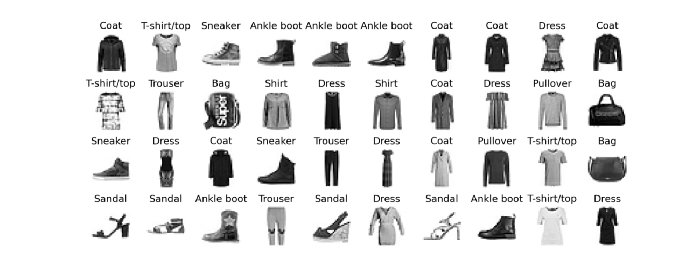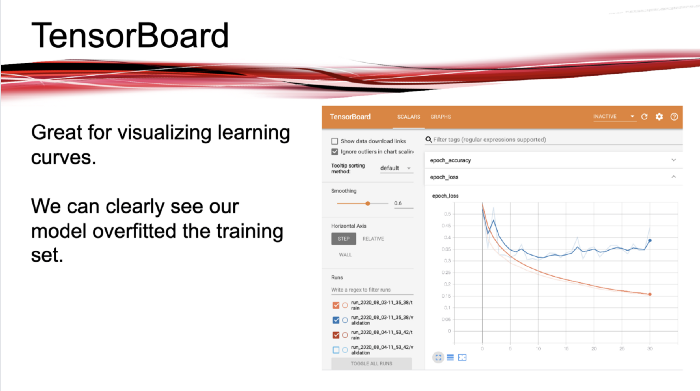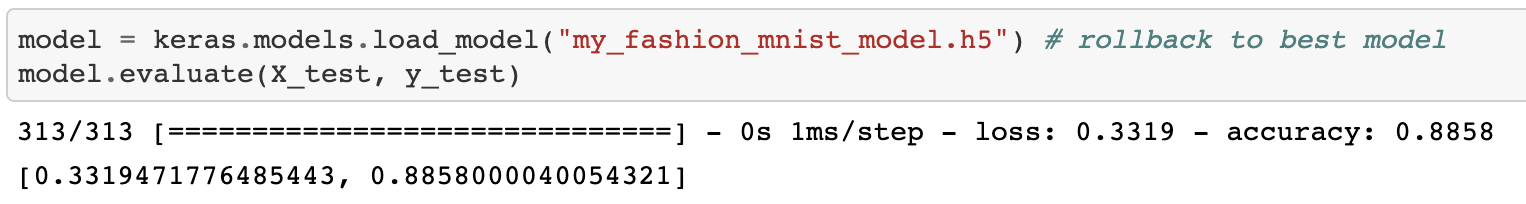# 深度学习的学习率调节实践

### 多层感知器### Keras模型

import os
import matplotlib.pyplot as plt
import numpy as np
import pandas as pd

PROJECT_ROOT_DIR = "."
IMAGES_PATH = os.path.join(PROJECT_ROOT_DIR, "images")
os.makedirs(IMAGES_PATH, exist_ok=True)

def save_fig(fig_id, tight_layout=True, fig_extension="png", resolution=300):
path = os.path.join(IMAGES_PATH, fig_id + "." + fig_extension)
print("Saving figure", fig_id)
if tight_layout:
plt.tight_layout()
plt.savefig(path, format=fig_extension, dpi=resolution)
import tensorflow as tf
from tensorflow import keras

(X_train, y_train), (X_test, y_test) = keras.datasets.fashion_mnist.load_data()

X_train.shape

X_train.dtype

X_valid, X_train = X_train[:5000] / 255.0, X_train[5000:] / 255.0
y_valid, y_train = y_train[:5000], y_train[5000:]
X_test = X_test / 255.0

class_names = ["T-shirt/top", "Trouser", "Pullover", "Dress", "Coat",
"Sandal", "Shirt", "Sneaker", "Bag", "Ankle boot"]

n_rows = 4
n_cols = 10
plt.figure(figsize=(n_cols * 1.2, n_rows * 1.2))
for row in range(n_rows):
for col in range(n_cols):
index = n_cols * row + col
plt.subplot(n_rows, n_cols, index + 1)
plt.imshow(X_train[index], cmap="binary", interpolation="nearest")
plt.axis('off')
plt.title(class_names[y_train[index]], fontsize=12)
save_fig('fashion_mnist_plot', tight_layout=False)
plt.show()model = keras.models.Sequential([
keras.layers.Flatten(input_shape=[28,28]),
keras.layers.Dense(300, activation="relu"),
keras.layers.Dense(100, activation="relu"),
keras.layers.Dense(10, activation="softmax")
])

• 首先，我们创建了一个Sequential模型，它是神经网络中最简单的Keras模型，它只由一堆按顺序连接的层组成。

• 接下来，我们构建第一层并将其添加到模型中。它是一个Flatten层，其目的是将每个输入图像转换成一个1D数组：如果它接收到输入数据X，则计算X.reshape（-1，1）。因为它是模型的第一层，所以应该指定输入形状。或者，你可以添加keras.layers.InputLayer作为第一层，设置其input_shape=[28,28]

• 下一步，我们添加一个300个神经元的隐藏层，并指定它使用ReLU激活函数。每一个全连接层管理自己的权重矩阵，包含神经元与其输入之间的所有连接权重。它还管理一个偏置向量，每个神经元一个。

• 然后我们添加了第二个100个神经元的隐藏层，同样使用ReLU激活函数。

• 最后，我们使用softmax激活函数添加了一个包含10个神经元的输出层（因为我们执行的分类是每个类都是互斥的）。

### 使用回调

K = keras.backend

class ExponentialLearningRate(keras.callbacks.Callback):
def __init__(self, factor):
self.factor = factor
self.rates = []
self.losses = []
def on_batch_end(self, batch, logs):
self.rates.append(K.get_value(self.model.optimizer.lr))
self.losses.append(logs["loss"])
K.set_value(self.model.optimizer.lr, self.model.optimizer.lr * self.factor)

model.compile(loss="sparse_categorical_crossentropy",
optimizer=keras.optimizers.SGD(lr=1e-3),
metrics=["accuracy"])
expon_lr = ExponentialLearningRate(factor=1.005)

history = model.fit(X_train, y_train, epochs=1,
validation_data=(X_valid, y_valid),
callbacks=[expon_lr])

plt.plot(expon_lr.rates, expon_lr.losses)
plt.gca().set_xscale('log')
plt.hlines(min(expon_lr.losses), min(expon_lr.rates), max(expon_lr.rates))
plt.axis([min(expon_lr.rates), max(expon_lr.rates), 0, expon_lr.losses])
plt.xlabel("Learning rate")
plt.ylabel("Loss")
save_fig("learning_rate_vs_loss")keras.backend.clear_session()
np.random.seed(42)
tf.random.set_seed(42)
model = keras.models.Sequential([
keras.layers.Flatten(input_shape=[28, 28]),
keras.layers.Dense(300, activation="relu"),
keras.layers.Dense(100, activation="relu"),
keras.layers.Dense(10, activation="softmax")
])
model.compile(loss="sparse_categorical_crossentropy",
optimizer=keras.optimizers.SGD(lr=2e-1),
metrics=["accuracy"])

### 使用TensorBoard进行可视化

TensorBoard是一个很好的交互式可视化工具，你可以使用它查看训练期间的学习曲线、比较学习曲线、可视化计算图、分析训练统计数据、查看模型生成的图像，可视化复杂的多维数据投影到三维和自动聚类，等等！这个工具是在安装TensorFlow时自动安装的，所以你应该已经安装了。

root_logdir = os.path.join(os.curdir, "my_logs")

def get_run_logdir():
import time
run_id = time.strftime("run_%Y_%m_%d-%H_%M_%S")
return os.path.join(root_logdir, run_id)

run_logdir = get_run_logdir() # 例如, './my_logs/run_2020_07_31-15_15_22'

Keras api提供了一个TensorBoard()回调函数。TensorBoard()回调函数负责创建日志目录,并在训练时创建事件文件和编写摘要(摘要是一种二进制数据记录用于创建可视化TensorBoard)。

early_stopping_cb = keras.callbacks.EarlyStopping(patience=20)
checkpoint_cb = keras.callbacks.ModelCheckpoint("my_fashion_mnist_model.h5", save_best_only=True)
tensorboard_cb = keras.callbacks.TensorBoard(run_logdir)

history = model.fit(X_train, y_train, epochs=100,
validation_data=(X_valid, y_valid),
callbacks=[early_stopping_cb, checkpoint_cb, tensorboard_cb])

%load_ext tensorboard
%tensorboard — logdir=./my_logs — port=6004### 结论• 使用Keras的Sequential API构建深度mlp。

• 通过按指数增长学习率，绘制损失图，并找到损失重新出现的点，来找到最佳学习率。

• 构建深度学习模型时的最佳实践，包括使用回调和使用TensorBoard可视化学习曲线。

#### 额外的资源

https://www.tensorflow.org/tensorboard/get_started

http://panchuang.net/

sklearn机器学习中文官方文档：
http://sklearn123.com/

http://docs.panchuang.net/